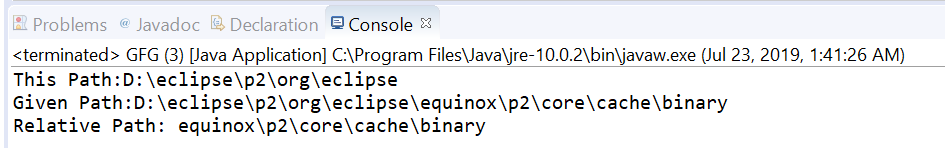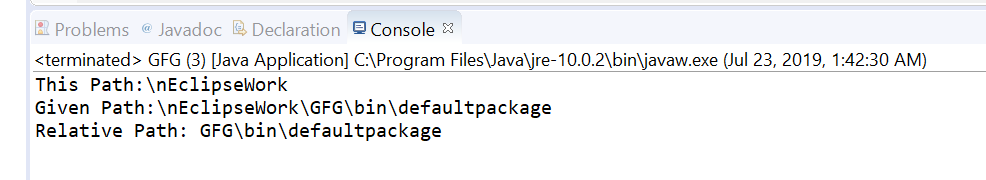Related Articles
Path relativize() method in Java with Examples
• Last Updated : 28 Jul, 2019

The relativize(Path other) method of java.nio.file.Path used to create a relative path between this path and a given path as a parameter.
Relativization is the inverse of resolution. This method creates a relative path that when resolved against this path object, yields a path that helps us to locate the same file as the given path.

For example, if this path is “/dir1/dir2” and the given path as a parameter is “/dir1/dir2/dir3/file1” then this method will construct a relative path “dir3/file1”. Where this path and the given path do not have a root component, then a relative path can be constructed.

If anyone of the paths has a root component then the relative path cannot be constructed. When both paths have a root component then it is implementation-dependent if a relative path can be constructed. If this path and the given path are equal then an empty path is returned.

Syntax:

```Path relativize(Path other)
```

Parameters: This method accepts a one parameter other which is the the path to relativize against this path.

Return value: This method returns the resulting relative path, or an empty path if both paths are equal.

Exception: This method throws IllegalArgumentException if other is not a Path that can be relativized against this path.

Below programs illustrate relativize() method:
Program 1:

 `// Java program to demonstrate ` `// java.nio.file.Path.relativize() method ` ` `  `import` `java.nio.file.Path; ` `import` `java.nio.file.Paths; ` `public` `class` `GFG { ` `    ``public` `static` `void` `main(String[] args) ` `    ``{ ` ` `  `        ``// create objects of Path ` `        ``Path path ` `            ``= Paths.get(``"D:\\eclipse\\p2"` `                        ``+ ``"\\org\\eclipse"``); ` `        ``Path passedPath ` `            ``= Paths.get(``"D:\\eclipse\\p2"` `                        ``+ ``"\\org\\eclipse\\equinox\\p2\\core"` `                        ``+ ``"\\cache\\binary"``); ` ` `  `        ``// print paths ` `        ``System.out.println(``"This Path:"` `                           ``+ path); ` `        ``System.out.println(``"Given Path:"` `                           ``+ passedPath); ` ` `  `        ``// call relativize() to create ` `        ``// a relative path ` `        ``Path relativize ` `            ``= path.relativize(passedPath); ` ` `  `        ``// print result ` `        ``System.out.println(``"Relative Path: "` `                           ``+ relativize); ` `    ``} ` `} `

Output:Program 2:

 `// Java program to demonstrate ` `// java.nio.file.Path.relativize() method ` ` `  `import` `java.nio.file.Path; ` `import` `java.nio.file.Paths; ` `public` `class` `GFG { ` `    ``public` `static` `void` `main(String[] args) ` `    ``{ ` ` `  `        ``// create objects of Path ` `        ``Path path ` `            ``= Paths.get(``"\\nEclipseWork"``); ` `        ``Path passedPath ` `            ``= Paths.get(``"\\nEclipseWork\\GFG"` `                        ``+ ``"\\bin\\defaultpackage"``); ` ` `  `        ``// print paths ` `        ``System.out.println(``"This Path:"` `                           ``+ path); ` `        ``System.out.println(``"Given Path:"` `                           ``+ passedPath); ` ` `  `        ``// call relativize() ` `        ``// to create a relative path ` `        ``Path relativize ` `            ``= path.relativize(passedPath); ` ` `  `        ``// print result ` `        ``System.out.println(``"Relative Path: "` `                           ``+ relativize); ` `    ``} ` `} `

Output:Attention reader! Don’t stop learning now. Get hold of all the important Java Foundation and Collections concepts with the Fundamentals of Java and Java Collections Course at a student-friendly price and become industry ready.

My Personal Notes arrow_drop_up
Recommended Articles
Page :